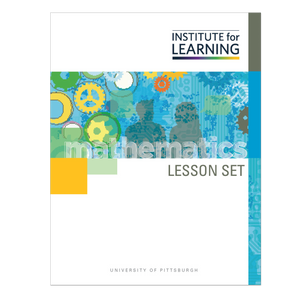Regular price \$6.25 \$0.00 Unit price per

In this lesson set, students extend their previous understanding of addition and subtraction of positive numbers to include negative numbers.

Through engaging in the tasks in this lesson set, students will:

• add opposite numbers and determine that their sum is always 0;
• make conjectures about sums and differences of positive and negative numbers based on observed patterns;
• develop an understanding of addition and subtraction as movement along the number line; and
• articulate the algorithm for adding and subtracting integers.

Standards: 7.NS.A.1, 7.NS.A.1.A, 7.NS.A.1.B, 7.NS.A.1.C, 7.NS.A.1.D, 7.NS.A.3